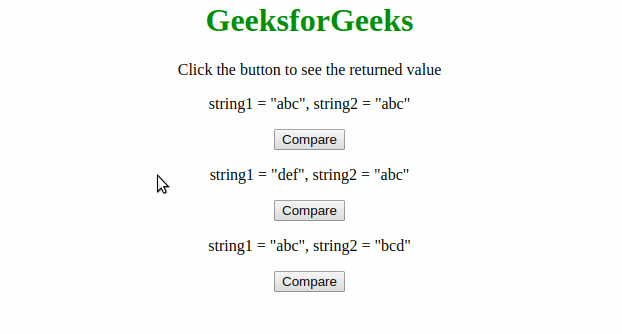# Is there anything similar to PHP strcmp() function in JavaScript ?

• Last Updated : 27 Apr, 2020

String comparison function is available in many languages like strcmp() in PHP, C, C++, (<, >, ==, !=) operators in Python etc. In JavaScript, to compare two string we use localCompare() method. The localCompare() method compares two strings in the present or current locale. It returns an integer to indicate whether the strings are equal, or, whether a string comes before or after of another string in sorted order.

Syntax

Hey geek! The constant emerging technologies in the world of web development always keeps the excitement for this subject through the roof. But before you tackle the big projects, we suggest you start by learning the basics. Kickstart your web development journey by learning JS concepts with our JavaScript Course. Now at it's lowest price ever!

```string1.localCompare(string2)
```

Return Value:  The localCompare() method returns

•  0, if the two strings: string1 and string2, are equal
• 1, if string1 is sorted after string2
• -1, if string1 is sorted before string2

Example:

## JavaScript

 ```<``html``>`` ` `<``head``>``    ``<``title``>``        ``Similar in JavaScript like PHP strcmp() Function``    ````    ``<``style``>``        ``h1 {``            ``color: green;``        ``}``    `````` ` `<``body``>``    ``<``center``>``        ``<``h1``>GeeksforGeeks``        ``<``p``>``            ``Click the button to see the returned value``        ```` ` `        ``<``p``>string1 = "abc", string2 = "abc"``        ``<``button` `onclick``=``"Comparison1()"``>``            ``Compare``        ```` ` `        ``<``p``>string1 = "def", string2 = "abc"``        ``<``button` `onclick``=``"Comparison2()"``>``            ``Compare``        ```` ` `        ``<``p``>string1 = "abc", string2 = "bcd"``        ``<``button` `onclick``=``"Comparison3()"``>``          ``Compare``        ```` ` `        ``<``p` `id``=``"compare"``>``    ```` ` `    ``<``script``>``        ``function Comparison1() {``            ``var string1 = "abc";``            ``var string2 = "abc";``            ``var return_val = string1.localeCompare(string2);``            ``document.getElementById("compare").innerHTML = ``              ``return_val;``        ``}`` ` `        ``function Comparison2() {``            ``var string1 = "def";``            ``var string2 = "abc";``            ``var return_val = string1.localeCompare(string2);``            ``document.getElementById("compare").innerHTML = ``              ``return_val;``        ``}`` ` `        ``function Comparison3() {``            ``var string1 = "abc";``            ``var string2 = "def";``            ``var return_val = string1.localeCompare(string2);``            ``document.getElementById("compare").innerHTML = ``              ``return_val;``        ``}``    `````` ` ``

Output:My Personal Notes arrow_drop_up